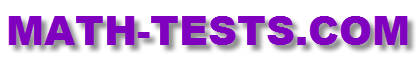#Are you looking for a 2nd grade math test that your kids or students can take online? On our website, we offer many free math tests and quizzes designed to assess student knowlege of various mathematical concepts taught in second grade.

These online tests are designed to work on computers, laptops, iPads, and other tablets. There is no need to download any app for these activities.

In this interactive test, kids will add different two-digit numbers (with and without regrouping.)

Subtracting Two-Digit Numbers
In this quiz, students will subtract two-digit numbers by using a variety of strategies.

Add Multiples of 10 Math Test
Mentally add multiples of 10 in this online math activity.

Interactive Place Value Activity
In this online quiz, 2nd grade students will use models, words, and numbers in expanded form to represent numbers to up to 1,000.

Money Quiz
Practice counting to find the value of pennies, nickels, dimes, and quarters, and different groups of coins.

Addition Sentences for Pictures Math Test
Use addition sentences to describe adding objects modeled in picture form in this math acvtivity.